# ML Aggarwal Solutions for Class 6 Maths Chapter 14 Mensuration

ML Aggarwal Solutions for Class 6 Maths Chapter 14 Mensuration are provided here. These ML Aggarwal Solutions are most valuable and assist students in learning complex and challenging topics. The solutions serve as an important study tool when it comes to preparing for the exam. Hence, students are suggested to solve all the questions provided in the ML Aggarwal Solutions for Class 6 Maths Chapter 14 to overcome mistakes while answering the questions.

Chapter 14 – Mensuration deals with length, area, perimeter and volume of different kinds of shapes both 2D and 3D. Mensuration is a formula oriented topic. There are specific formulas for various parameters, we put the values in these Mensuration formulas and get the desired answers. ML Aggarwal Solutions are provided in the pdf format, students can easily download the PDF from the below mentioned links.

## Download the PDF of ML Aggarwal Solutions for Class 6 Maths Chapter 14 Mensuration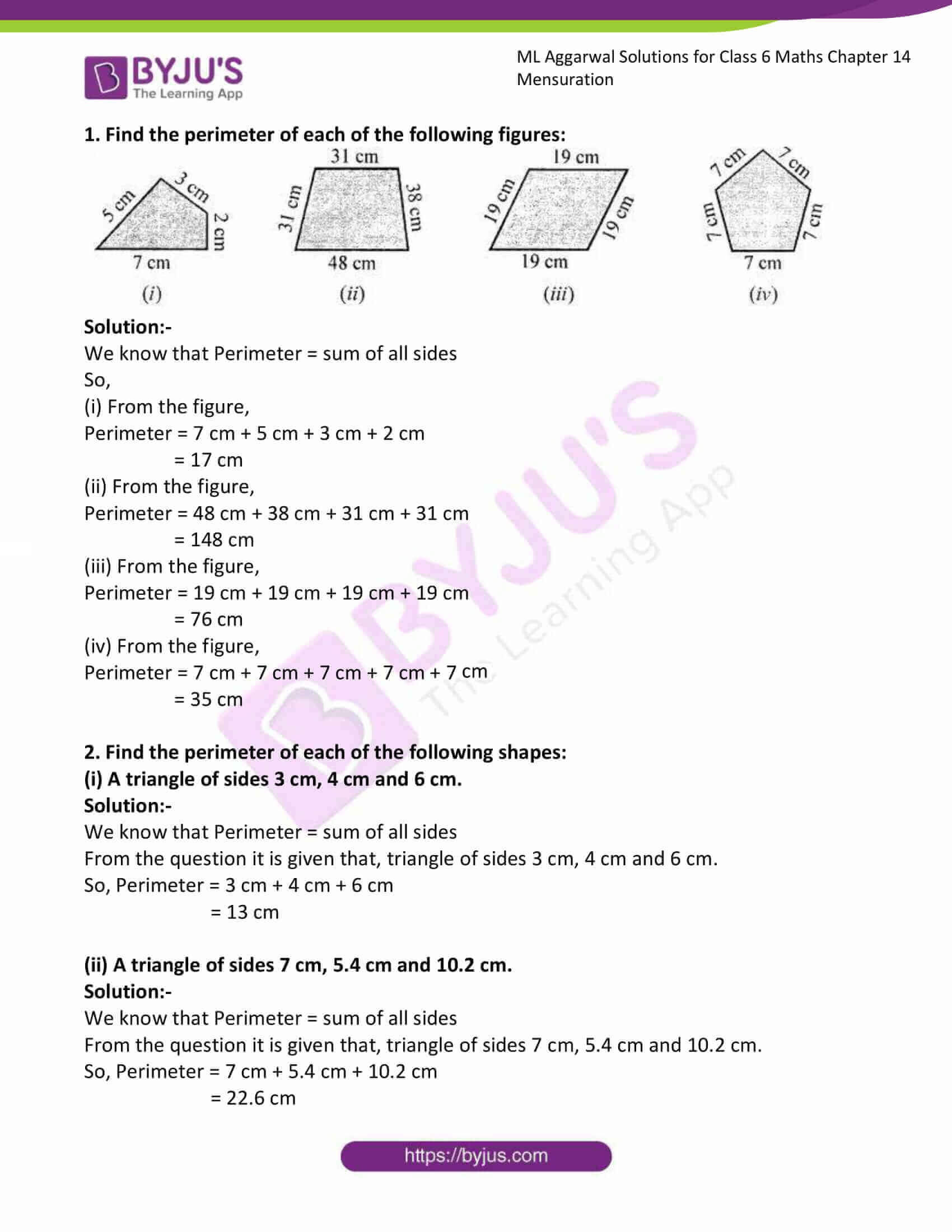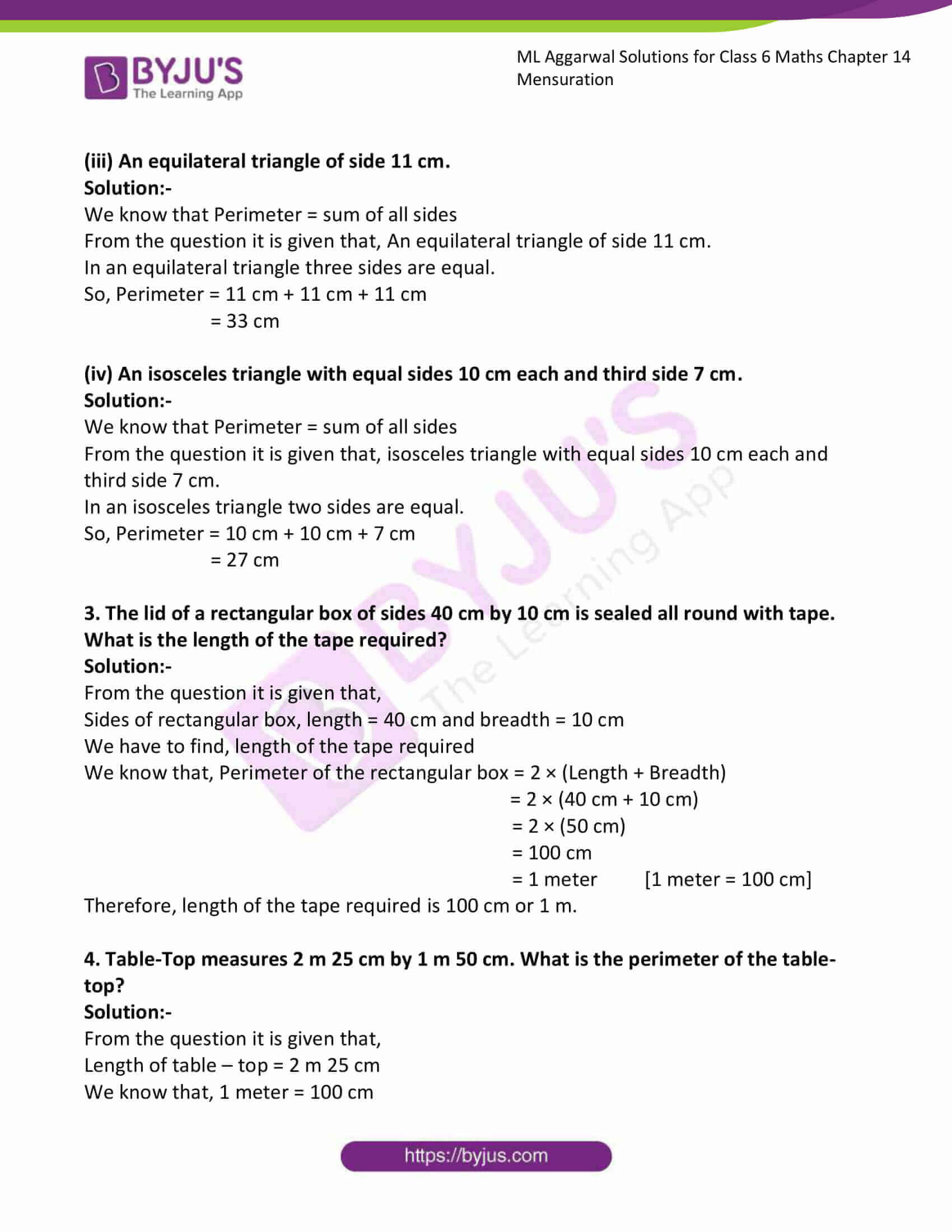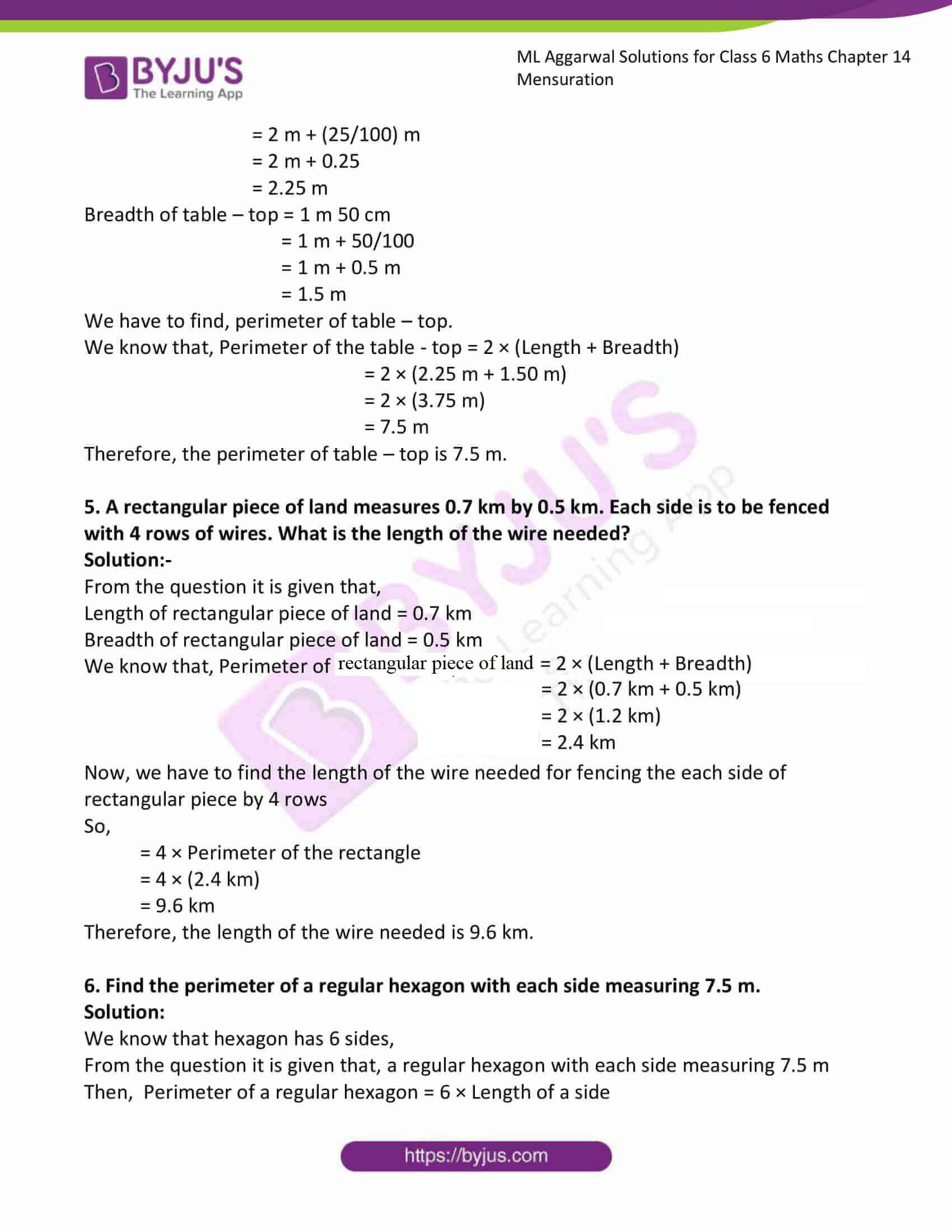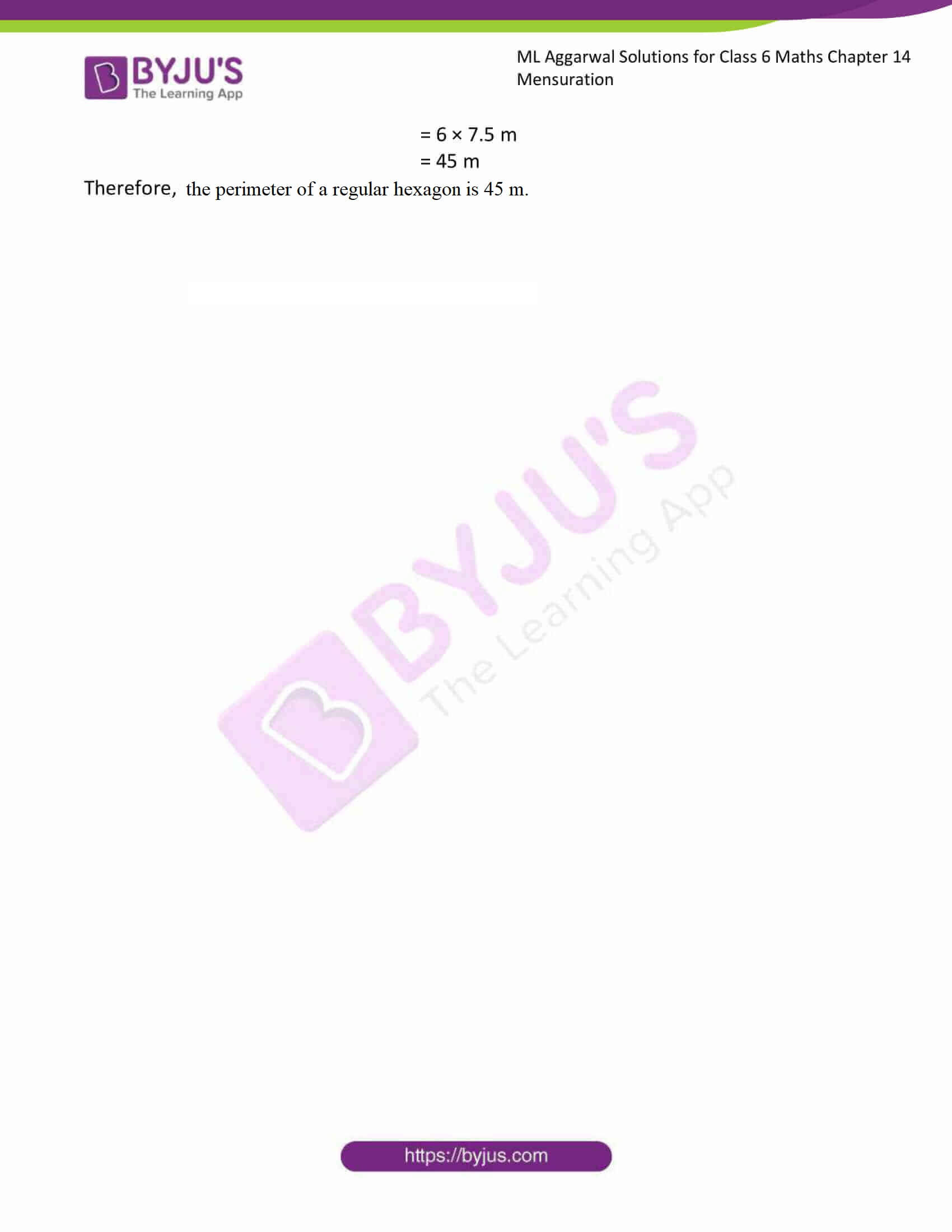### Access answers to ML Aggarwal Solutions for Class 6 Maths Chapter 14 Mensuration

1. Find the perimeter of each of the following figures: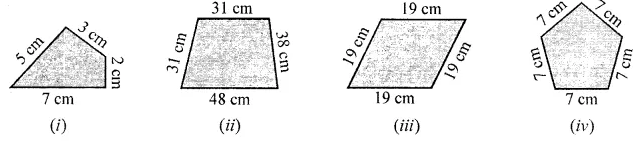Solution:-

We know that Perimeter = sum of all sides

So,

(i) From the figure,

Perimeter = 7 cm + 5 cm + 3 cm + 2 cm

= 17 cm

(ii) From the figure,

Perimeter = 48 cm + 38 cm + 31 cm + 31 cm

= 148 cm

(iii) From the figure,

Perimeter = 19 cm + 19 cm + 19 cm + 19 cm

= 76 cm

(iv) From the figure,

Perimeter = 7 cm + 7 cm + 7 cm + 7 cm + 7 cm

= 35 cm

2. Find the perimeter of each of the following shapes:
(i) A triangle of sides 3 cm, 4 cm and 6 cm.

Solution:-

We know that Perimeter = sum of all sides

From the question it is given that, triangle of sides 3 cm, 4 cm and 6 cm.

So, Perimeter = 3 cm + 4 cm + 6 cm

= 13 cm

(ii) A triangle of sides 7 cm, 5.4 cm and 10.2 cm.

Solution:-

We know that Perimeter = sum of all sides

From the question it is given that, triangle of sides 7 cm, 5.4 cm and 10.2 cm.

So, Perimeter = 7 cm + 5.4 cm + 10.2 cm

= 22.6 cm

(iii) An equilateral triangle of side 11 cm.

Solution:-

We know that Perimeter = sum of all sides

From the question it is given that, An equilateral triangle of side 11 cm.

In an equilateral triangle three sides are equal.

So, Perimeter = 11 cm + 11 cm + 11 cm

= 33 cm

(iv) An isosceles triangle with equal sides 10 cm each and third side 7 cm.

Solution:-

We know that Perimeter = sum of all sides

From the question it is given that, isosceles triangle with equal sides 10 cm each and third side 7 cm.

In an isosceles triangle two sides are equal.

So, Perimeter = 10 cm + 10 cm + 7 cm

= 27 cm

3. The lid of a rectangular box of sides 40 cm by 10 cm is sealed all round with tape. What is the length of the tape required?
Solution:-

From the question it is given that,

Sides of rectangular box, length = 40 cm and breadth = 10 cm

We have to find, length of the tape required

We know that, Perimeter of the rectangular box = 2 × (Length + Breadth)

= 2 × (40 cm + 10 cm)

= 2 × (50 cm)

= 100 cm

= 1 meter [1 meter = 100 cm]

Therefore, length of the tape required is 100 cm or 1 m.

4. Table-Top measures 2 m 25 cm by 1 m 50 cm. What is the perimeter of the table-top?
Solution:-

From the question it is given that,

Length of table – top = 2 m 25 cm

We know that, 1 meter = 100 cm

= 2 m + (25/100) m

= 2 m + 0.25

= 2.25 m

Breadth of table – top = 1 m 50 cm

= 1 m + 50/100

= 1 m + 0.5 m

= 1.5 m

We have to find, perimeter of table – top.

We know that, Perimeter of the table – top = 2 × (Length + Breadth)

= 2 × (2.25 m + 1.50 m)

= 2 × (3.75 m)

= 7.5 m

Therefore, the perimeter of table – top is 7.5 m.

5. A rectangular piece of land measures 0.7 km by 0.5 km. Each side is to be fenced with 4 rows of wires. What is the length of the wire needed?
Solution:-

From the question it is given that,

Length of rectangular piece of land = 0.7 km

Breadth of rectangular piece of land = 0.5 km

We know that, Perimeter of rectangular piece of land = 2 × (Length + Breadth)

= 2 × (0.7 km + 0.5 km)

= 2 × (1.2 km)

= 2.4 km

Now, we have to find the length of the wire needed for fencing the each side of rectangular piece by 4 rows

So,

= 4 × Perimeter of the rectangle

= 4 × (2.4 km)

= 9.6 km

Therefore, the length of the wire needed is 9.6 km.

6. Find the perimeter of a regular hexagon with each side measuring 7.5 m.
Solution:

We know that hexagon has 6 sides,

From the question it is given that, a regular hexagon with each side measuring 7.5 m

Then, Perimeter of a regular hexagon = 6 × Length of a side

= 6 × 7.5 m

= 45 m

Therefore, the perimeter of a regular hexagon is 45 m.